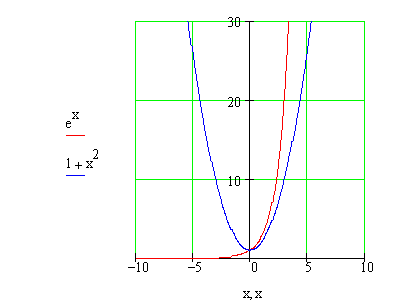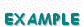Calculus on Demand at Dartmouth College Lecture 4 | Index | Lecture 6 Lecture 5## Resources

Math 3 Course Syllabus
Practice Exams

# Contents

In this lecture we consider an extended application of the ideas of the first four lectures, namely, modeling AIDS data.

### Quick Question

How many solutions does the equation ex − x2 − 1 = 0 have?### Outline

Outline for Case Study: Modeling with Elementary Functions

### Textbook

Sec. 1.7, Case Study: Modeling with Elementary Functions

### Quiz

Case Study: Modeling with Elementary Functions Quiz

### Examples

•A succession of forest fires has been decimating the countryside. A table of data is available, giving the days elapsed and acres destroyed. Two functions have been suggested to model the data. Which one is a better fit?
•After examining the data in the table, it is suggested that since the amount of damage is increasing so quickly, perhaps an exponential function would be a better fit. Is this true? Find the best-fit exponential function and determine if it does in fact model the data more precisely. Use the two polynomials suggested in example 1 to predict the number of acres
•Use the two polynomials suggested in example 1 to predict the number of acres destroyed after 30, 40, and 50 days. It turns out that after 30 days, the number of acres destroyed was 25100. What was the percentage error in each of your estimates? How does this reflect on your choice of modeling function?

### Videos

Lecture 4 | Index | Lecture 6